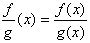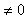Dividing

When dividing two functions,, remember that g(x)since division by zero is undefined. To divide a polynomial by a monomial, divide each term of the polynomial by the monomial. Remember to watch the signs of the terms when dividing and that a number divided by itself is one, do not cancel these terms.

Listen